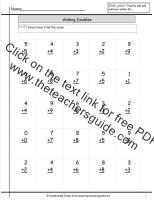# The Teacher's Guide-Free Worksheets, SMARTboard templates, and lesson plans for teachers.

###Doubles Addition Facts Worksheet

25 doubles problems.

###Doubles Addition Facts Worksheet

50 doubles problems.

###Doubles Addition Facts Worksheet

100 doubles problems.

###Doubles Addition Facts Worksheet Timed

25 doubles problems timed.

###Doubles Plus One Addition Facts Worksheet

25 doubles plus one problems.

###Doubles Plus One Addition Facts Worksheet

50 doubles plus one problems.

###Doubles Plus One Addition Facts Worksheet

100 doubles plus one problems.

###Doubles Plus One Addition Facts Worksheet Timed

25 doubles plus one problems timed.

###Doubles Plus One Intro Addition Facts Worksheet

Introduction to doubles plus one problems.

###Adding Ten Addition Facts Worksheet

Adding ten worksheet with word problems.

###Sums of Five, Six, and Seven Addition Facts Worksheet

50 problems sums of five, six and seven.

###Sums of Five, Six, and Seven Addition Facts Worksheet

100 problems sums of five, six and seven.

###Sums of Five, Six, and Seven Addition Facts Worksheet

25 problems sums of five, six and seven timed.

###Sums of Eight and Nine Addition Facts Worksheet

25 problems sums of eight and nine.

###Sums of Eight and Nine Addition Facts Worksheet

50 problems sums of eight and nine.

###Sums of Eight and Nine Addition Facts Worksheet

100 problems sums of eight and nine.

###Sums of Eight and Nine Addition Facts Worksheet

25 problems sums of eight and nine timed.

###Sums of Ten Addition Facts Worksheet

Finding the sums of ten worksheet.  This worksheet includes sums of ten cues at the top.  20 problems.

###Sums of Ten Addition Facts Worksheet

Finding the sums of ten worksheet. This worksheet includes sums of ten cues at the top.  50 problems.

###Sums of Ten Addition Facts Worksheet

Finding the sums of ten worksheet. No cues.  21 problems.

###Sums of Ten Addition Facts Worksheet

Finding the sums of ten worksheet. No cues. 50 problems.

###Sums of Ten Addition Facts Worksheet

Finding the sums of ten worksheet with word problems..